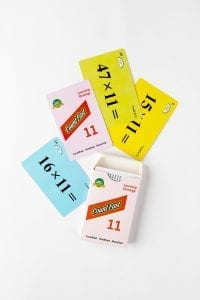# CountFast 11 Home Activities

####OVERVIEW & PURPOSE

Week 7 of the 3rd Grade CountFast program focuses on strategies to multiply two and three-digit numbers by 11.  Spend 15 minutes each day on one of the activities listed in this module.  Card decks should go home with students each day for additional practice with a parent at home. Each week, a new deck is introduced, and the previous deck is for the student to keep at home for continued practice.

#### EDUCATION STANDARDS

1. NCTM Standard: develop a sense of whole numbers and represent and use them in flexible ways, including relating, composing, and decomposing numbers
2. Math.Content.3.OA.B.5
Apply properties of operations as strategies to multiply and divide.

#### OBJECTIVES

1. Mentally multiply two-digit numbers by 11.
2. Mentally multiply three-digit numbers by 11.
DAY 1 – 5:This week, your child is learning how to multiply any two or three-digit number by 11.  On Days 1 and 2, we will focus on two-digit numbers.  On Days 3 and 4, we will focus on three-digit numbers.  Day 5 will be a review of both.  The cards you will need for each day’s practice are:Day 1 – YellowDay 2 – BlueDay 3 – Pink

Day 4 – Green

Day 5 – All (review)

Here are the strategies we are learning for mental calculation with 11:

(The trick is to split the digits of the original number you are multiplying by 11 and in the middle space add those digits together to give you the answer. Remember you may need to carry a number forward.)  See these examples.

Two-Digits:

34 x 11 = 3 (3+4) 4                65 x 11 = 6 (6+5) 5

= 374                                                                     carry forward = 6 (11) 5

Carry 1 forward and add to 6 = 715

Three-Digits:

Start by splitting the first and last digits apart and leave two spaces between.  Add the first 2 digits to fill the first space, and add the back 2 digits to fill the second space.

135 x 11 = 1 (1+3)(3+5) 5                                  302 x 11 = 3 (3+0)(0+2) 2

= 1485                                                             = 3322

Stay SharpFree flash card decks to keep kids entertained and sharp!

To help you keep your kids math sharp during this crisis, we're giving you 3 free CountFast flash card decks for just \$6 in shipping.  Just have them take the quick quiz for their grade at the link below and you'll get a discount code to use on any three decks.